JMSLTM Numerical Library 7.2.0
com.imsl.stat

## Class ARMAMaxLikelihood

• All Implemented Interfaces:
Serializable

```public class ARMAMaxLikelihood
extends Object
implements Serializable```
Computes maximum likelihood estimates of parameters for an ARMA model with p and q autoregressive and moving average terms respectively.

`ARMAMaxLikelihood` computes estimates of parameters for a nonseasonal ARMA model given a sample of observations,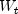, for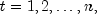where`z.length`. The class is derived from the maximum likelihood estimation algorithm described by Akaike, Kitagawa, Arahata and Tada (1979), and the XSARMA routine published in the TIMSAC-78 Library.

The stationary time serieswith mean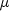can be represented by the nonseasonal autoregressive moving average (ARMA) model by the following relationship: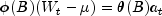where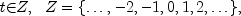B is the backward shift operator defined by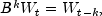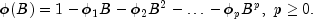and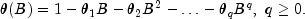The `ARMAMaxLikelihood` class estimates the AR coefficients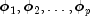and the MA coefficients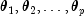using maximum likelihood estimation.

`ARMAMaxLikelihood` checks the initial estimates for both the autoregressive and moving average coefficients to ensure that they represent a stationary and invertible series respectively.

If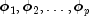are the initial estimates for a stationary series then all (complex) roots of the following polynomial will fall outside the unit circle: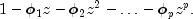If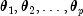are initial estimates for an invertible series then all (complex) roots of the polynomial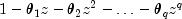will fall outside the unit circle.

By default, the order of the lags for the autoregressive terms is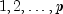and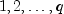for the moving average terms. However, this cannot be overridden.

Initial values for the `AR` and `MA` coefficients can be supplied via the `setAR` and `setMA` methods. Otherwise, initial estimates are computed internally by the method of moments. The class computes the roots of the associated polynomials. If the `AR` estimates represent a nonstationary series, `ARMAMaxLikelihood` issues a warning message and replaces the intial `AR` estimates with initial values that are stationary. If the `MA` estimates represent a noninvertible series, a terminal error is issued and new initial values must be sought.

`ARMAMaxLikelihood` also validates the final estimates of the `AR` coefficients to ensure that they too represent a stationary series. This is done to guard against the possibility that the internal log-likelihood optimizer converged to a nonstationary solution. If nonstationary estimates are encountered, a fatal error message is issued.

For model selection, the `ARMA` model with the minimum value for AIC might be preferred,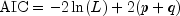, where L is the value of the maximum likelihood function evaluated at the parameter estimates.

`ARMAMaxLikelihood` can also handle white noise processes, i.e.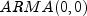processes.

The Java Logging API can be used to trace the execution of `ARMAMaxLikelihood`. The name of this logger is `com.imsl.stat.ARMAMaxLikelihood`. Accumulated levels of detail correspond to Java's FINE, FINER, and FINEST logging levels with FINE yielding the smallest amount of information and FINEST yielding the most. The levels of output yield the following:

 Level Output `FINE` A message on entering and exiting method `compute`. `FINER` All of the messages in `FINE`, a message entering and exiting the method `compute`, plus the final computations. `FINEST` All of the messages in `FINER`, and a table of the computed weights and their gradient values.

Example, Serialized Form
• ### Nested Class Summary

Nested Classes
Modifier and Type Class and Description
`static class ` `ARMAMaxLikelihood.InitialMAException`
The initial values for the moving average parameters are not invertible.
`static class ` `ARMAMaxLikelihood.NonInvertibleException`
The solution is noninvertible.
`static class ` `ARMAMaxLikelihood.NonStationaryException`
The solution is nonstationary.
• ### Constructor Summary

Constructors
Constructor and Description
```ARMAMaxLikelihood(int p, int q, double[] z)```
Constructor for `ARMAMaxLikelihood`.
• ### Method Summary

Methods
Modifier and Type Method and Description
`void` `compute()`
Computes the exact maximum likelihood estimates for the autoregressive and moving average parameters of an ARMA time series
`double[][]` `forecast(int nForecast)`
Returns forecasts for lead times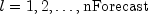at origins `z.length-backwardOrigin-1+j` where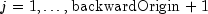.
`double[]` `getAR()`
Returns the final autoregressive parameter estimates.
`int` `getBackwardOrigin()`
Returns the current value for forecasting backward origin.
`double` `getConfidence()`
Returns the confidence level used for calculating deviations in `getDeviations`.
`double` `getConstant()`
Returns the estimate for the constant parameter in the ARMA series.
`double[]` `getDeviations()`
Returns the deviations from each forecast used for calculating the forecast confidence limits.
`double[]` `getForecast(int nForecast)`
Returns forecasts
`double[]` `getGradients()`
Returns the gradients for the final parameter estimates.
`double` `getGradientTolerance()`
Returns the gradient tolerance for the convergence algorithm.
`double` `getInnovationVariance()`
Returns the estimated innovation variance of this series.
`double` `getLikelihood()`
Returns the final estimate for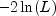, where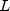is equal to the likelihood function evaluated using the final parameter estimates.
`double[]` `getMA()`
Returns the final moving average parameter estimates.
`int` `getMaxIterations()`
Returns the maximum number of iterations.
`double` `getMean()`
Returns the mean used to center the time series.
`int` `getP()`
Returns the number of autoregressive terms in the ARMA model
`double[]` `getPsiWeights()`
Returns the psi weights used for calculating forecasts from the infinite order moving average form of the ARMA model.
`int` `getQ()`
Returns the number of moving average terms in the ARMA model
`double[]` `getResiduals()`
Returns the current values of the vector of residuals.
`double[]` `getTimeSeries()`
Returns the time series used to construct `ARMAMaxLikelihood`.
`double` `getTolerance()`
Returns the tolerance for the convergence algorithm.
`boolean` `isInvertible(double[] ma)`
Tests whether the coefficients in `ma` are invertible
`boolean` `isStationary(double[] ar)`
Tests whether the coefficients in `ar` are stationary.
`void` `setAR(double[] ar)`
Sets the initial values for the autoregressive terms to the `p` values in `ar`.
`void` `setBackwardOrigin(int backwardOrigin)`
Sets the maximum backward origin.
`void` `setConfidence(double confidence)`
Sets the confidence level for calculating confidence limit deviations returned from `getDeviations()`.
`void` `setConstant(double constant)`
Sets the initial value for the constant term in the ARMA model.
`void` `setGradientTolerance(double gradientTolerance)`
Sets the tolerance for the convergence algorithm.
`void` `setMA(double[] ma)`
Sets the initial values for the moving average terms to the `q` values in `ma`.
`void` `setMaxIterations(int maxIterations)`
Sets the maximum number of iterations.
`void` `setMean(double wMean)`
Sets the mean used for centering the series.
`void` `setTolerance(double tolerance)`
Sets the tolerance for the convergence algorithm.
• ### Methods inherited from class java.lang.Object

`clone, equals, finalize, getClass, hashCode, notify, notifyAll, toString, wait, wait, wait`
• ### Constructor Detail

• #### ARMAMaxLikelihood

```public ARMAMaxLikelihood(int p,
int q,
double[] z)
throws ARMA.MatrixSingularException,
ARMA.TooManyCallsException,
ARMA.IncreaseErrRelException,
ARMA.NewInitialGuessException,
ARMA.IllConditionedException,
ARMA.TooManyITNException,
ARMA.TooManyFcnEvalException,
ARMA.TooManyJacobianEvalException```
Constructor for `ARMAMaxLikelihood`.
Parameters:
`p` - An `int` scalar equal to the number of autoregressive (AR) parameters.
`q` - An `int` scalar equal to the number of moving average (MA) parameters.
`z` - A `double` array containing the time series.
Throws:
`ARMA.MatrixSingularException` - is thrown if the input matrix is singular.
`ARMA.TooManyCallsException` - is thrown if the number of calls to the function has exceeded the maximum number of iterations times the number of moving average (MA) parameters + 1.
`ARMA.IncreaseErrRelException` - is thrown if the bound for the relative error is too small.
`ARMA.NewInitialGuessException` - is thrown if the iteration has not made good progress.
`ARMA.IllConditionedException` - is thrown if the problem is ill-conditioned.
`ARMA.TooManyITNException` - is thrown if the maximum number of iterations is exceeded.
`ARMA.TooManyFcnEvalException` - is thrown if the maximum number of function evaluations exceeded.
`ARMA.TooManyJacobianEvalException` - is thrown if the maximum number of Jacobian evaluations is exceeded.
• ### Method Detail

• #### compute

```public void compute()
throws ARMAMaxLikelihood.NonStationaryException,
ARMAMaxLikelihood.NonInvertibleException,
ARMAMaxLikelihood.InitialMAException```
Computes the exact maximum likelihood estimates for the autoregressive and moving average parameters of an ARMA time series
Throws:
`ARMAMaxLikelihood.NonStationaryException` - is thrown if the final maximum likelihood estimates for the time series are nonstationary.
`ARMAMaxLikelihood.NonInvertibleException` - is thrown if the final maximum likelihood estimates for the time series are noninvertible.
`ARMAMaxLikelihood.InitialMAException` - is thrown if the initial values provided for the moving average terms using `setMA` are noninvertible. In this case, ```ARMAMaxLikelihood ``` terminates and does not compute the time series estimates.
• #### forecast

```public double[][] forecast(int nForecast)
throws ARMAMaxLikelihood.NonStationaryException,
ARMAMaxLikelihood.NonInvertibleException,
ARMAMaxLikelihood.InitialMAException,
ARMA.MatrixSingularException,
ARMA.TooManyCallsException,
ARMA.IncreaseErrRelException,
ARMA.NewInitialGuessException,
ARMA.IllConditionedException,
ARMA.TooManyITNException,
ARMA.TooManyFcnEvalException,
ARMA.TooManyJacobianEvalException```
Returns forecasts for lead timesat origins `z.length-backwardOrigin-1+j` where.
Parameters:
`nForecast` - An `int` scalar equal to the number of requested forecasts
Returns:
a `double` matrix of dimensions of `nForecast` by `backwardOrigin + 1` containing the forecasts. The forecasts are for lead timesat origins `z.length-backwardOrigin-1+j` where.
Throws:
`ARMAMaxLikelihood.NonStationaryException` - is thrown if the final maximum likelihood estimates for the time series are nonstationary.
`ARMAMaxLikelihood.NonInvertibleException` - is thrown if the final maximum likelihood estimates for the time series are noninvertible.
`ARMAMaxLikelihood.InitialMAException` - is thrown if the initial values provided for the moving average terms using `setMA` are noninvertible. In this case, ```ARMAMaxLikelihood ``` terminates and does not compute the time series estimates.
`ARMA.MatrixSingularException` - is thrown if the input matrix is singular.
`ARMA.TooManyCallsException` - is thrown if the number of calls to the function has exceeded the maximum number of iterations times the number of moving average (MA) parameters + 1.
`ARMA.IncreaseErrRelException` - is thrown if the bound for the relative error is too small.
`ARMA.NewInitialGuessException` - is thrown if the iteration has not made good progress.
`ARMA.IllConditionedException` - is thrown if the problem is ill-conditioned.
`ARMA.TooManyITNException` - is thrown if the maximum number of iterations is exceeded.
`ARMA.TooManyFcnEvalException` - is thrown if the maximum number of function evaluations exceeded.
`ARMA.TooManyJacobianEvalException` - is thrown if the maximum number of Jacobian evaluations is exceeded.
• #### getAR

`public double[] getAR()`
Returns the final autoregressive parameter estimates.
Returns:
A `double` array of length `p` containing the final autoregressive parameter estimates.
• #### getBackwardOrigin

`public int getBackwardOrigin()`
Returns the current value for forecasting backward origin.
Returns:
A `double` scalar value representing the current value of `backwardOrigin`
• #### getConfidence

`public double getConfidence()`
Returns the confidence level used for calculating deviations in `getDeviations`.
Returns:
A `double` scalar value of `confidence` used for computing the `(1-confidence)*100%` forecast confidence interval.
• #### getConstant

`public double getConstant()`
Returns the estimate for the constant parameter in the ARMA series.
Returns:
A `double` scalar equal to the estimate for the constant parameter in the ARMA series.
• #### getDeviations

`public double[] getDeviations()`
Returns the deviations from each forecast used for calculating the forecast confidence limits.
Returns:
A `double` array of length `backwardOrigin+nForecast` containing the deviations for calculating forecast confidence intervals. The confidence level is specified in `confidence`. By default, `confidence`= 0.95.
• #### getForecast

```public double[] getForecast(int nForecast)
throws ARMAMaxLikelihood.NonStationaryException,
ARMAMaxLikelihood.NonInvertibleException,
ARMAMaxLikelihood.InitialMAException,
ARMA.MatrixSingularException,
ARMA.TooManyCallsException,
ARMA.IncreaseErrRelException,
ARMA.NewInitialGuessException,
ARMA.IllConditionedException,
ARMA.TooManyITNException,
ARMA.TooManyFcnEvalException,
ARMA.TooManyJacobianEvalException```
Returns forecasts
Parameters:
`nForecast` - An input `int` representing the number of requested forecasts beyond the last value in the series.
Returns:
A `double` array containing the `nForecast+backwardOrigin` forecasts. The first `backwardOrigin` forecasts are one-step ahead forecasts for the last `backwardOrigin` values in the series. The next `nForecast` values in the returned series are forecasts for the next values beyond the series.
Throws:
`ARMAMaxLikelihood.NonStationaryException` - is thrown if the final maximum likelihood estimates for the time series are nonstationary.
`ARMAMaxLikelihood.NonInvertibleException` - is thrown if the final maximum likelihood estimates for the time series are noninvertible.
`ARMAMaxLikelihood.InitialMAException` - is thrown if the initial values provided for the moving average terms using `setMA` are noninvertible. In this case, ```ARMAMaxLikelihood ``` terminates and does not compute the time series estimates.
`ARMA.MatrixSingularException` - is thrown if the input matrix is singular.
`ARMA.TooManyCallsException` - is thrown if the number of calls to the function has exceeded the maximum number of iterations times the number of moving average (MA) parameters + 1.
`ARMA.IncreaseErrRelException` - is thrown if the bound for the relative error is too small.
`ARMA.NewInitialGuessException` - is thrown if the iteration has not made good progress.
`ARMA.IllConditionedException` - is thrown if the problem is ill-conditioned.
`ARMA.TooManyITNException` - is thrown if the maximum number of iterations is exceeded.
`ARMA.TooManyFcnEvalException` - is thrown if the maximum number of function evaluations exceeded.
`ARMA.TooManyJacobianEvalException` - is thrown if the maximum number of Jacobian evaluations is exceeded.

`public double[] getGradients()`
Returns the gradients for the final parameter estimates.
Returns:
A `double` array of length `p+q` containing the gradients of the final parameter estimates.

`public double getGradientTolerance()`
Returns the gradient tolerance for the convergence algorithm.
Returns:
A `double` scalar specifying the value used for the gradient tolerance.
• #### getInnovationVariance

`public double getInnovationVariance()`
Returns the estimated innovation variance of this series.
Returns:
A `double` scalar equal to the estimated innovation variance for the time series.
• #### getLikelihood

```public double getLikelihood()
throws ARMAMaxLikelihood.NonStationaryException,
ARMAMaxLikelihood.NonInvertibleException,
ARMAMaxLikelihood.InitialMAException```
Returns the final estimate for, whereis equal to the likelihood function evaluated using the final parameter estimates.
Returns:
A `double` scalar equal to the log likelihood function,.
Throws:
`ARMAMaxLikelihood.NonStationaryException` - is thrown if the final maximum likelihood estimates for the time series are nonstationary.
`ARMAMaxLikelihood.NonInvertibleException` - is thrown if the final maximum likelihood estimates for the time series are noninvertible.
`ARMAMaxLikelihood.InitialMAException` - is thrown if the initial values provided for the moving average terms using `setMA` are noninvertible. In this case, ```ARMAMaxLikelihood ``` terminates and does not compute the time series estimates.
• #### getMA

`public double[] getMA()`
Returns the final moving average parameter estimates.
Returns:
A `double` array of length `q` containing the final moving average parameter estimates.
• #### getMaxIterations

`public int getMaxIterations()`
Returns the maximum number of iterations.
Returns:
An `int` scalar containing the maximum number of iterations allowed in the nonlinear equation solver used in both the method of moments and least-squares algorithms. Default: `maxIterations` = 300.
• #### getMean

`public double getMean()`
Returns the mean used to center the time series.
Returns:
A `double` scalar specifying the value to used for centering the time series.
• #### getP

`public int getP()`
Returns the number of autoregressive terms in the ARMA model
Returns:
An `int` scalar value of `p`, the number of autoregressive terms in the ARMA model.
• #### getPsiWeights

`public double[] getPsiWeights()`
Returns the psi weights used for calculating forecasts from the infinite order moving average form of the ARMA model.
Returns:
A `double` array of length `nForecast` containing the psi weights of the infinite order moving average form of the model.
• #### getQ

`public int getQ()`
Returns the number of moving average terms in the ARMA model
Returns:
An `int` scalar value of `q`, the number of moving average terms in the ARMA model.
• #### getResiduals

`public double[] getResiduals()`
Returns the current values of the vector of residuals.
Returns:
A `double` array of length `backwardOrigin` containing the residuals for the last `backwardOrigin` values in the time series. The `compute` and either the `forecast` or `getForecast` methods must be called before calling this method.
• #### getTimeSeries

`public double[] getTimeSeries()`
Returns the time series used to construct `ARMAMaxLikelihood`.
Returns:
A `double` containing the values of the time series passed to the class constructor.
• #### getTolerance

`public double getTolerance()`
Returns the tolerance for the convergence algorithm.
Returns:
A `double` scalar containing the value of the tolerance used during maximum likelihood estimation.
• #### isInvertible

`public boolean isInvertible(double[] ma)`
Tests whether the coefficients in `ma` are invertible
Parameters:
`ma` - A `double` array containing the coefficients for the moving average terms in an ARMA model.
Returns:
A `boolean` scalar equal to `true` if the coefficients in `ma` are invertible and `false` otherwise.
• #### isStationary

`public boolean isStationary(double[] ar)`
Tests whether the coefficients in `ar` are stationary.
Parameters:
`ar` - A `double` array containing the coefficients for the autoregressive terms in an ARMA model
Returns:
A `boolean` scalar equal to `true` if the coefficients in `ar` are stationary and ```false ``` otherwise.
• #### setAR

`public void setAR(double[] ar)`
Sets the initial values for the autoregressive terms to the `p` values in `ar`.
Parameters:
`ar` - An input `double` array of length `p` containing the initial values for the autoregressive terms. If this method is not called, initial values are computed by method of moments in the `ARMA` class.
• #### setBackwardOrigin

`public void setBackwardOrigin(int backwardOrigin)`
Sets the maximum backward origin.
Parameters:
`backwardOrigin` - An `int` scalar specifying the maximum backward origin used in forecasting. `backwardOrigin` must be greater than or equal to 0 and less than or equal to `z.length - Math.max(p, q)`. Default: `backwardOrigin = 0`.
• #### setConfidence

`public void setConfidence(double confidence)`
Sets the confidence level for calculating confidence limit deviations returned from `getDeviations()`.
Parameters:
`confidence` - a `double` scalar specifying the confidence level used in computing forecast confidence intervals. Typical choices for `confidence` are 0.90, 0.95, and 0.99. `confidence` must be greater than 0.0 and less than 1.0. Default: `confidence = 0.95`.
• #### setConstant

`public void setConstant(double constant)`
Sets the initial value for the constant term in the ARMA model.
Parameters:
`constant` - A `double` scalar specifying the initial value for the constant term in the ARMA model. By default, the constant term is initially estimated using ARMA method of moments estimation.

`public void setGradientTolerance(double gradientTolerance)`
Sets the tolerance for the convergence algorithm.
Parameters:
`gradientTolerance` - A `double` scalar specifying the the tolerance used for numerically estimating the gradient by differences. Default: `gradientTolerance = 1e-04`.
• #### setMA

`public void setMA(double[] ma)`
Sets the initial values for the moving average terms to the `q` values in `ma`.
Parameters:
`ma` - A `double` array of length `q` containing the initial values for the moving average terms. If this method is not called, initial values are computed by method of moments in the `ARMA` class.
Throws:
`ARMAMaxLikelihood.InitialMAException` - is thrown if the number of initial values provided for the moving average terms using `setMA` is not equal to `q`.
• #### setMaxIterations

```public void setMaxIterations(int maxIterations)
throws IllegalArgumentException```
Sets the maximum number of iterations.
Parameters:
`maxIterations` - An `int` scalar specifying the maximum number of iterations allowed in the nonlinear equation solver used in both the method of moments and least-squares algorithms. Default: `maxIterations` = 300.
Throws:
`IllegalArgumentException`
• #### setMean

`public void setMean(double wMean)`
Sets the mean used for centering the series.
Parameters:
`wMean` - A `double` scalar specifying the value to use for centering the time series. By default the series is centered using the mean of the series.
• #### setTolerance

`public void setTolerance(double tolerance)`
Sets the tolerance for the convergence algorithm.
Parameters:
`tolerance` - A `double` scalar specifying the value to use for the convergence tolerance. Default: `tolerance = 2.220446049e-016`.
JMSLTM Numerical Library 7.2.0